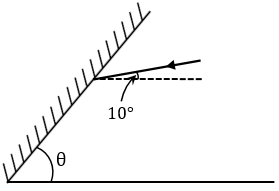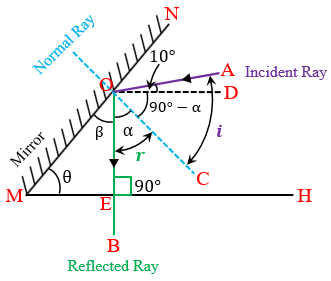"
">

# A plane mirror is inclined at an angle theta (╬Ė) with horizontal as shown in the figure. If a ray incident from 10Ōü░ above horizontal becomes vertical after reflection from this mirror, then find theta (╬Ė)."Given:

The angle of incident above the horizontal = 10⁰

To find : Angle theta (θ).

Solution:

According to the laws of reflection, we know that angle of incidence $(i)$ is equal to the angle of reflection $(r)$ . $(\angle i=\angle r)$

Here,

$Angle\ AOC(\angle i)=Angle\ BOC(\angle r)$

Hence,

$( 90-\alpha ) +10=\alpha$         $[ \because \angle i=( 90-\alpha ) ,\ \angle AOD=10,\ and\ \angle r=\alpha ]$

$90+10=\alpha+\alpha$

$2\alpha=100$

$\alpha=\frac {100}{2}$

$\alpha=50$

Now,

$\alpha+\beta=90$                 $[ \because \angle COM=90]$

Putting the value of $\alpha$ in the above equation we get-

$50+\beta=90$

$\beta=90-50$

$\beta=40$

In $\angle MOE$

$\angle MOE+\angle EMO+\angle MEO=180$    $[ \because\ sum\ of\ measures\ of\ angles\ in\ triangle\ are\ 180 ]$

$\beta+\theta+90=180$                  $[\angle MEO=90, because\ the\ refracted\ ray\ OB\ is\ perpendiculat\ to\ the\ horizontal\ MH]$

Putting the value of $\beta$ in the above equation we get-

$40+\theta+90=180$

$\theta=180-130$

$\theta=50$

Thus, the value of  theta$(\theta)$ is 50⁰.

Updated on: 10-Oct-2022

2K+ Views